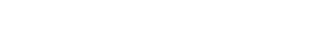# Greedy algorithm python – Coin change problemA greedy python algorithm (greedy algorithm python) greedily selects the best choice at every step. He hopes that these choices lead to the optimal overall solution to the problem. So, a greedy algorithm does not always give the best solution. However in many problems this is the case.

## Greedy Algorithm: Introduction

The problem of giving change is formulated as follows. How to return a given sum with a minimum of coins and banknotes?

Here is an example in python of the resolution of the problem:

If we consider the Euro monetary system without the cents we have the whole

EURO = (1, 2, 5, 10, 20, 50, 100, 200, 500)

## Greedy algorithm python : Coin change problem

Now, to give change to an x ​​value of using these coins and banknotes, then we will check the first element in the array. And if it’s greater than x, we move on to the next element. Otherwise let’s keep it. Now, after taking a valuable coin or bill from the array of coinAndBill [i], the total value x we ​​need to do will become x – coinAndBill [i].

Here is the associated greedy python algorithm:

```def renduMonnaieGlouton(x):
pieceEtBillets = [500,200,100,50,20,10,5,2,1]
i = 0

while(x>0):
if(pieceEtBillets[i] > x):
i = i+1
else:
print(str(pieceEtBillets[i]))
x -= pieceEtBillets[i];

renduMonnaieGlouton(33)#Exemple sur 33 euros```

The output for 33 euro is then:

```20
10
2
1```

Another example with 55 euro of greedy python algorithm:

```def renduMonnaieGlouton(x):
pieceEtBillets = [500,200,100,50,20,10,5,2,1]
i = 0

while(x>0):
if(pieceEtBillets[i] > x):
i = i+1
else:
print(str(pieceEtBillets[i]))
x -= pieceEtBillets[i];

renduMonnaieGlouton(55)#Exemple sur 55 euros```

Output :

```50
5```

## Conclusion

The problem of giving change is NP-difficult relative to the number of coins and notes of the monetary system considered (euro in this example). To go further, we can demonstrate that for certain so-called canonical money systems, the use of a greedy algorithm is optimal. A monetary system is said to be canonical if for any sum s the greedy algorithm leads to a minimal decomposition.

NP difficulty is the defining property of a class of problems informally “at least as difficult as the most difficult NP problems”.

A simple example of an NP-hard problem is the sum of subset problem. If P is different from NP then it is unlikely to find a polynomial time algorithm that exactly solves this problem.

## External links :

http://math.univ-lyon1.fr/irem/IMG/pdf/monnaie.pdf

http://www.dil.univ-mrs.fr/~gcolas/algo-licence/slides/gloutons.pdf

## Internal links python greedy algorithm :

https://128mots.com/index.php/category/python/

https://128mots.com/index.php/category/non-classe/